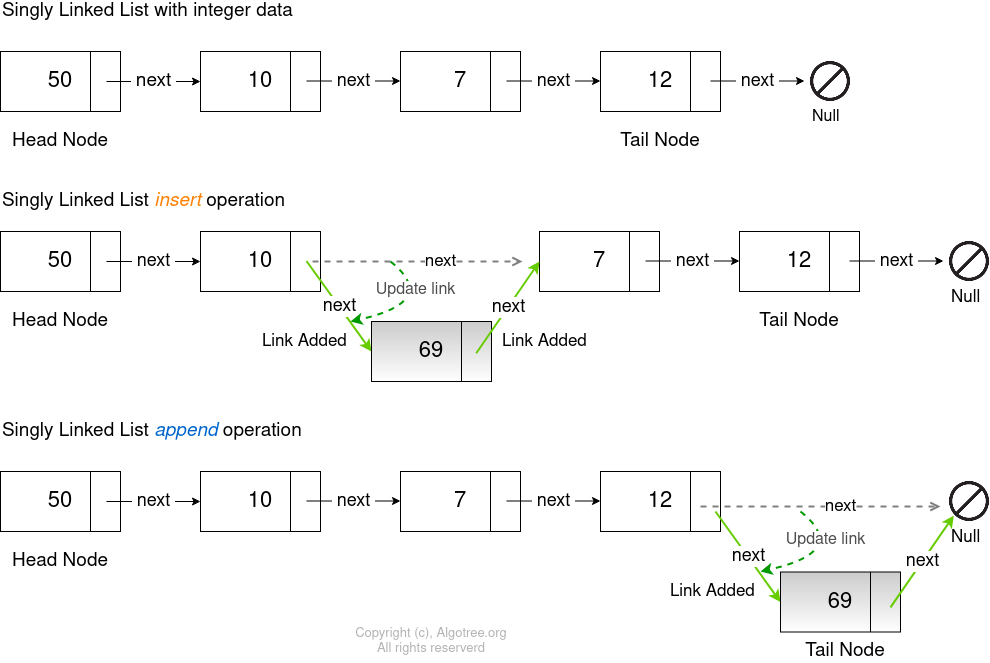Singly Linked List : Inserting, Appending and Freeing nodes.

Insert Operation
To insert into a singly-linked list, we need to know the node after which we need to insert a new_node.
1. Traverse the linked list and store the location of the node ( into current_node ) after which a new_node is to be inserted.
2. If the current_node is not Null then
Create a new_node that is to be inserted.
Store the location of the node that is next to the current_node i.e ( next_node = current . next )
Update the next link of the current_node by pointing it to the new_node.
Point the new_node’s next link to next_node.
Append Operation
To append to a singly-linked list,
1. Traverse the linked list till the end. Store the location of the last node into current_node.
Create a new_node to be appended.
Update the next link of the current_node by pointing it to the new_node.
Below is the example of an insert and append operationFree Operation
To free the singly linked list, we start from the head node and do the below
While the HEAD node of the singly linked list is not NULL.
a) Store the node next to HEAD in a temporary node.
c) Point the HEAD to the temporary node.

Time complexity for inserting a node : O ( N ), as the linked list has be to traversed to find the node after which a new node is to be inserted.
Time complexity for appending a node : O ( N ), as the linked list has be to traversed to reach the last node after which a new node is to be inserted.
Time complexity for freeing the linked list : O ( N ), as every node of the linked list has be to traversed before it is deleted.

Space complexity : O ( N ). N is the number of nodes in the linked list.

C++ program for inserting, appending and freeing the nodes of a singly linked list.

#include<iostream>

using namespace std;

class Node {

public:
int data;
Node* next;
Node (int arg_data) : data (arg_data), next (nullptr)
{}
};

public:

{}

// Insert a new node into the single linked list after the first found node.
void Insert (Node* head, int data, int after) {

// Traverse the link list till the node a node is found after
// which the data is to be insert
while (current->data != after) {
current = current->next;
}

if (current != nullptr) {
Node * new_node = new Node(data);
// Save the location of node after current in next_node link
Node * next_node = current->next;
// Point current's link to the new node to be inserted.
current->next = new_node;
// Point new node's link to the next node location previously stored.
new_node->next = next_node;
}
}

// Append a node to the singly linked list
void Append (Node* head, int data) {

while (current->next != nullptr) {
current = current->next;
}
Node* new_node = new Node(data);
current->next = new_node;
}

while (current != nullptr) {
cout << current->data << " ";
current = current->next;
}
}

}
}
};

int main() {

Node *node_3 = new Node(3);
Node *node_5 = new Node(5);
Node *node_7 = new Node(7);
Node *node_13 = new Node(13);

node_3->next = node_5;
node_5->next = node_7;
node_7->next = node_13;

int data, after, opt = 0;

while (opt != 3) {
cout << "\n\nOptions" << endl;
cout << "1. Insert" << endl;
cout << "2. Append" << endl;
cout << "3. Exit" << endl;
cout << "Enter Option : ";
cin >> opt;
switch (opt) {

case 1:
cout << "\nEnter new node (data) : ";
cin >> data;
cout << "After node : ";
cin >> after;
break;

case 2:
cout << "\nEnter new node (data) : ";
cin >> data;
break;

case 3:
cout << "Freeing the linked list." << endl;
break;
}
}
return 0;
}

Output

Options
1. Insert
2. Append
3. Exit
Enter Option : 1
Linked list 2 3 5 7 13
Enter new node (data) : 8
After node : 7
2 3 5 7 8 13

Options
1. Insert
2. Append
3. Exit
Enter Option : 1
Linked list 2 3 5 7 8 13
Enter new node (data) : 20
After node : 8
2 3 5 7 8 20 13

Options
1. Insert
2. Append
3. Exit
Enter Option : 2
Linked list 2 3 5 7 8 20 13
Enter new node (data) : 40
2 3 5 7 8 20 13 40

Options
1. Insert
2. Append
3. Exit
Enter Option : 2
Linked list 2 3 5 7 8 20 13 40
Enter new node (data) : 100
2 3 5 7 8 20 13 40 100

Options
1. Insert
2. Append
3. Exit
Enter Option : 3